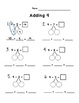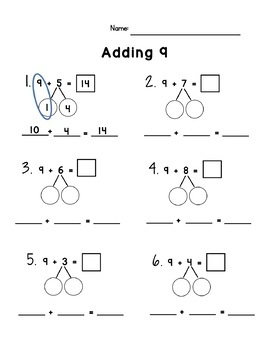# Adding 9 with Single DigitsSubject
Resource Type
File Type
PDF (1 MB|8 pages)
Standards
FREE
• Product Description
• Standards
This resource is intended to break down the mental process of adding 9 to single digits. There are 3 different thought processes included. When students have a conceptual understanding of what it means to add 9, their fact fluency will improve.
Fluently add and subtract within 20 using mental strategies. By end of Grade 2, know from memory all sums of two one-digit numbers.
Add and subtract within 20, demonstrating fluency for addition and subtraction within 10. Use strategies such as counting on; making ten (e.g., 8 + 6 = 8 + 2 + 4 = 10 + 4 = 14); decomposing a number leading to a ten (e.g., 13 - 4 = 13 - 3 - 1 = 10 - 1 = 9); using the relationship between addition and subtraction (e.g., knowing that 8 + 4 = 12, one knows 12 - 8 = 4); and creating equivalent but easier or known sums (e.g., adding 6 + 7 by creating the known equivalent 6 + 6 + 1 = 12 + 1 = 13).
Total Pages
8 pages